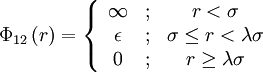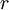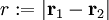Square shoulder model

The square shoulder model is given by$\Phi_{12}\left( r \right) = \left\{ \begin{array}{ccc} \infty & ; & r < \sigma \\ \epsilon & ; &\sigma \le r < \lambda \sigma \\ 0 & ; & r \ge \lambda \sigma \end{array} \right.$

where$\Phi_{12}(r)$ is the intermolecular pair potential,$\epsilon$ is the height of the shoulder,$r$ is the distance between site 1 and site 2$r := |\mathbf{r}_1 - \mathbf{r}_2|$, σ is the hard diameter and λ > 1.

.